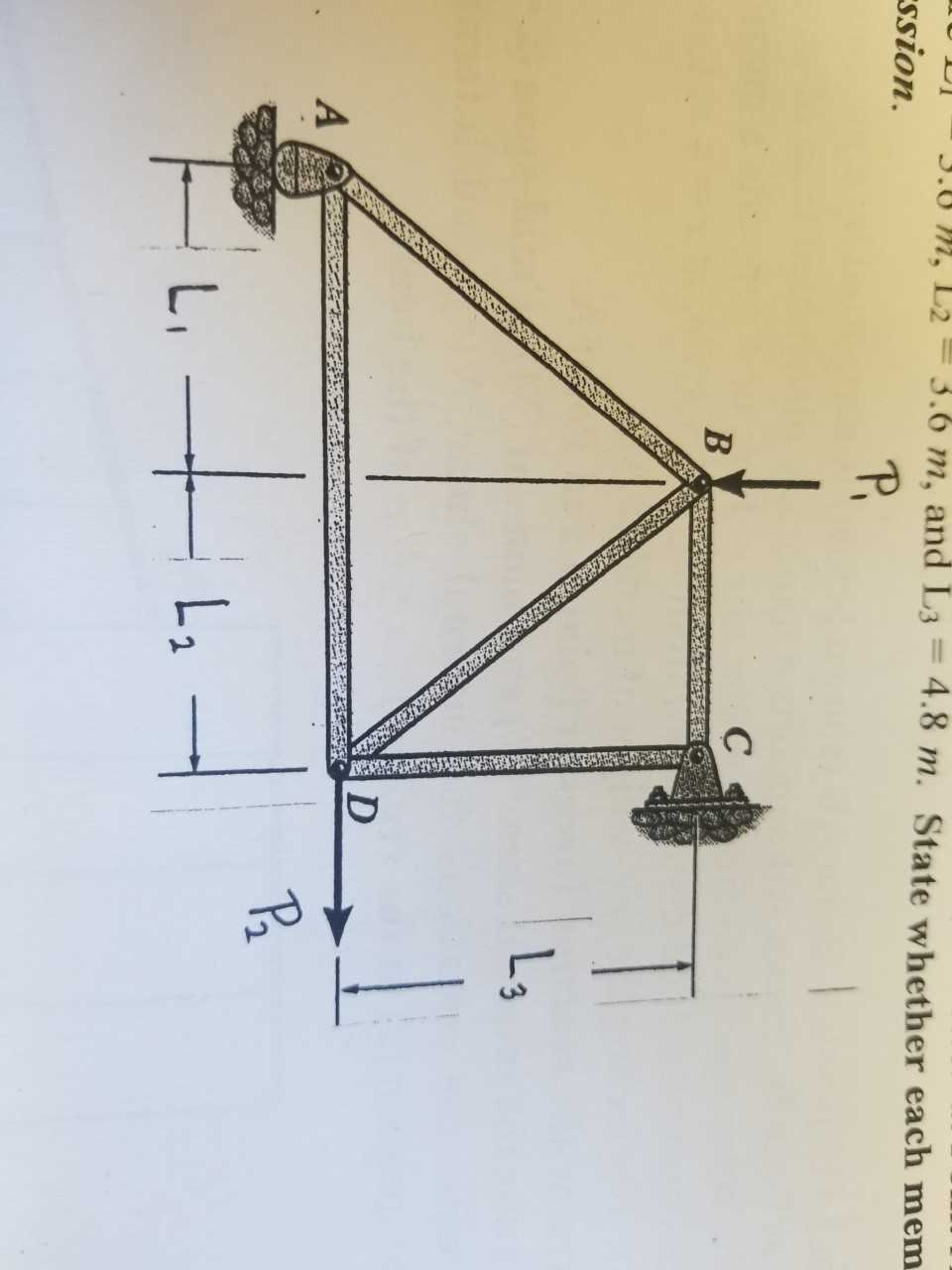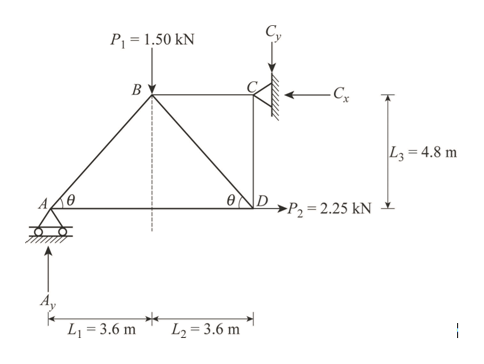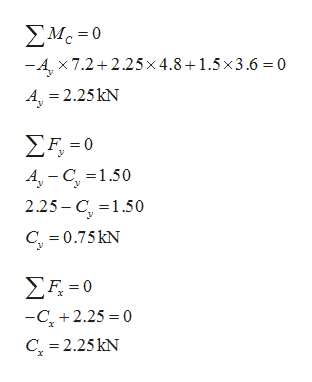Question
53 views

Determine the force in each member of the truss ABCD. The truss supports a vertical load of P1=1.50kN at Point B and a horzontal load P2=2.25kN at Point D. The truss is supported by a pin connection at Point C and a roller at Point A and the truss dimensions are L1=3.6m, L2=3.6m, and L3=4.8m. State whether the members are in tension or compression.help_outlineImage Transcriptionclose3.0 m, L2 3.6 m, and L3 4.8 m. State whether each mem ssion. P. C B Lg D A L2 fullscreen
check_circle

Step 1

Apply the sign conventions for calculating reactions, forces and moments using the three equations of equilibrium as shown below.

• The forces acting towards right side as positive and the forces acting towards left side as negative.
• The upward force as positive and the downward force as negative.
• The clockwise moment as negative and the counter clockwise moment as positive.

Show the free body diagram of the truss as shown in Figure 1.help_outlineImage TranscriptioncloseP 1.50 kN B -Cx |L3= 4.8 m OND >P,=2.25 kN A E L1 = 3.6 m L2= 3.6 m fullscreen
Step 2

Apply the Equations of Equilibirium as follows:help_outlineImage TranscriptioncloseΣΜ.-0 -A, x 7.2 2.25x 4.8+1.5x3.6 = 0 A,-2.25 Ν Σ-0 A-C 1.50 2.25- C 1.50 C 0.75kN ΣΕ-0 -C2.25 0 C 2.25kN fullscreen
Step 3

Calculate the value of the...

### Want to see the full answer?

See Solution

#### Want to see this answer and more?

Solutions are written by subject experts who are available 24/7. Questions are typically answered within 1 hour.*

See Solution
*Response times may vary by subject and question.
Tagged in

### Civil Engineering